### Day 76 - Volume/Arc Length - 05.07.15

Update(s)
• Unit 6 - Applications of Integration
• Area of a Region
• Volume of a Region: Disk Method
• Arc Length and Surface Area of a Region
• Unit 6 Test
• next Tuesday, May 12th
• Summative 3 Exam
• covers Units 5 and 6
• next Friday, May 15th
• Seniors Final Exam (optional)
• Monday, May 18th
• 8-12am
• Gym

Questions

Bell Ringer
• Posted on the board!

Review
• Prerequisites
• Area of a Region
• How can the area of a region be calculated using definite integrals?
• Sketch areas of regions between given equations.
• Calculate area of regions between equations using calculus.
• Activity
• Checkpoints
• Extra Practice

Lesson
• Volume
•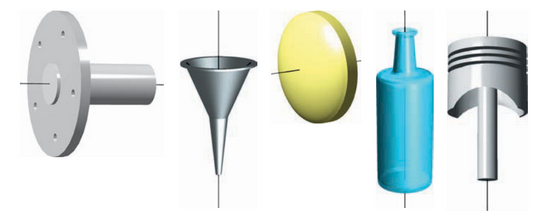• The Disk Method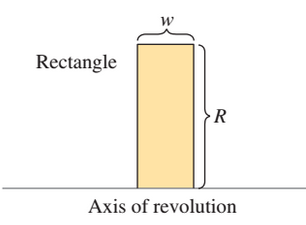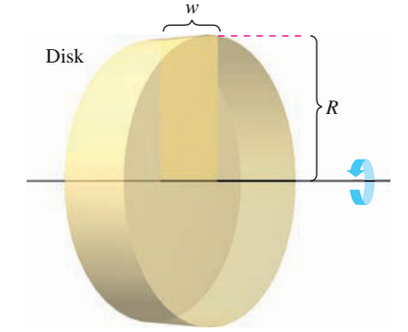• The Washer Method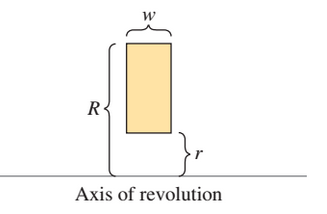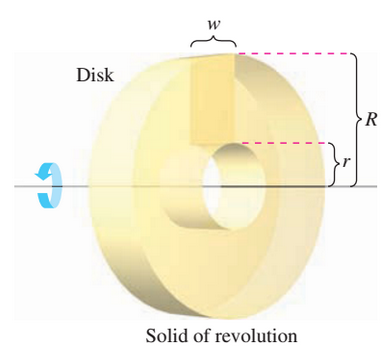Exit Ticket
• Posted on the board at the end of the block!

Lesson Objective(s)
• How can the volume of a solid of revolution be found?
Skills
1. Find volume using disk method.
2. Find volume using washer method.

#### In-Class Help Requests

Standard(s)
• APC.15​
• The student will use integration techniques and appropriate integrals to model physical, biological, and economic situations. The emphasis will be on using the integral of a rate of change to give accumulated change or on using the method of setting up an approximating Riemann sum and representing its limit as a definite integral. Specific applications will include
• a)​ the area of a region;
• b) ​the volume of a solid with known cross-section;
• c)​ the average value of a function; and
• d)​ the distance traveled by a particle along a line.